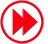# HDTV TECH

## An Eye for Color: Physics & Physiology Sidebars

Back To Basics
All wave phenomena, such as sound and light, exhibit several distinct characteristics. Among these characteristics are frequency and amplitude. Frequency is the rate at which something varies periodically over time. It is measured in cycles/second, a unit of measurement that is also called hertz (Hz), after Heinrich Hertz (1857–1894), a German physicist, engineer, and mathematician who was a pioneer in the study of electromagnetism. Thousands of cycles per second are called kilohertz (kHz), while millions of cycles per second are called megahertz (MHz).

Waves also exhibit two additional characteristics related to frequency: wavelength and speed. Wavelength is the distance between consecutive crests or troughs of the wave (see Fig.A). As you might imagine, speed is the rate at which the wave travels through space. In the case of sound and light, the speed in a given medium (e.g., air) is constant, regardless of the frequency or wavelength.

These three characteristics are related by the equation:

speed = frequencyxwavelength

If you apply a little algebra to this equation, you get two equivalent equations that sometimes come in handy, depending on the values you know and the value you want to calculate:

frequency = speed/wavelength

wavelength = speed/frequency

Amplitude is the "depth" or "height" of the wave (i.e., its maximum displacement from equilibrium; see Fig.A). A related characteristic is intensity, which is proportional to the square of the amplitude. For example, if the amplitude doubles, the intensity quadruples; if the amplitude increases by a factor of three, the intensity increases by a factor of nine.

The actual physical amplitude of these wave phenomena is very small, making it an unwieldy and impractical measurement for general use. Therefore, intensity is typically used to express the amplitude of sound and light. In addition, intensity is more directly related to our perception of the loudness of a sound or the brightness of a light source. The specific units of measurement for intensity are different for sound and light waves.—SWFig.A: Waves are defined by several characteristics, including wavelength and amplitude.

Nothing Comes Between Me And My Kelvins
You might think it a minor matter, but the precise expression of color temperature has been a point of some debate. Color temperature is derived from the absolute temperature scale developed by William Thomson, Baron Kelvin of Largs. As a result, it is tempting to express absolute temperature and color temperature in units of degrees Kelvin (°K), just as we express everyday temperature in degrees Celsius (°C) or degrees Fahrenheit (°F).

However, in 1960, the Eleventh General Conference of Weights and Measures decided to define the units of absolute temperature as kelvins in order to make this unit of measurement consistent with other units named after specific people. For example, units of power are called watts (W), after James Watt. Notice that when the unit is spelled out, the first letter is not capitalized. However, the abbreviation is capitalized in honor of the person for whom it is named. As a result, SGHT uses units of kelvins (K) to express color temperature.—SWARTICLE CONTENTSX